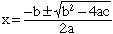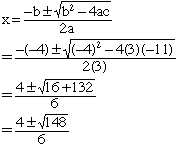Quandaries and Queries Name: Kathleen Who is asking: Other Level: Secondary Question: hi im having a problem trying to understand quadratic formulas can u please help me i have problems like 3x2 - 4x - 11 = 0 how do i work out that problem? i have the formula written down but it doesnt seem to register for me. Hi Kathleen, The quadratic formula states that if ax2 + bx + c = 0 thenLook at your equation 3x2 - 4x - 11 = 0 and compare it to the general equation above, ax2 + bx + c = 0 In your equation the coefficient of x2 is 3, in the gereral equation it is a. In your equation the coefficient of x is -4, in the gereral equation it is b. In your equation the constant term is -11, in the gereral equation it is c. Thus in the expression for x put a = 3, b = -4 and c = -11. That isThis will simplify further. Penny Go to Math Central